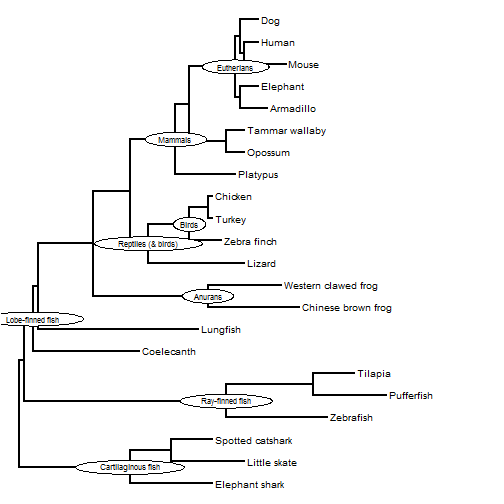## Tuesday, February 21, 2017

### Interactive node labeling using phytools

When it comes to labeling internal nodes on a phylogenetic tree in R, the function `nodelabels` in the ape package can pretty much do it all. Nonetheless, when I was contacted recently by a colleague I realized there was space for some additional functionality - specifically, in terms of allowing the user to interact with the plotting device to determine the location of said labels - something that is not currently possible using `ape::nodelabels` (so far as I can tell).

The code below consists of two different functions. The first returns the index of the closest node on the plotting device for a currently plotted phylogeny. The second writes a label to that node, with a few different options from `nodelabels`. Note that `getnode` could easily be combined with `ape::nodelabels` to take advantage of all the functionality of `nodelabels` but in an interactive context.

``````getnode<-function(...){
if(hasArg(env)) env<-list(...)\$env
else env<-get("last_plot.phylo",envir=.PlotPhyloEnv)
xy<-unlist(locator(n=1))
points(xy,xy)
d<-sqrt((xy-env\$xx)^2+(xy-env\$yy)^2)
ii<-which(d==min(d))
ii
}

labelnodes<-function(text,node=NULL,interactive=TRUE,
shape=c("circle","ellipse","rect"),...){
shape<-shape
if(hasArg(circle.exp)) circle.exp<-list(...)\$circle.exp
else circle.exp<-1.3
if(hasArg(rect.exp)) rect.exp<-list(...)\$rect.exp
else rect.exp<-1.6
if(hasArg(cex)) cex<-list(...)\$cex
else cex<-1
obj<-get("last_plot.phylo",envir=.PlotPhyloEnv)
h<-cex*strheight("A")
w<-cex*strwidth(text)
if(is.null(node)){
if(!interactive){
cat("No nodes provided. Setting interactive mode to TRUE.\n")
interactive<-TRUE
}
node<-vector(length=length(text))
}
for(i in 1:length(text)){
if(interactive){
cat(paste("Click on the node you would like to label ",
text[i],".\n",sep=""))
flush.console()
ii<-getnode(env=obj)
node[i]<-ii
} else ii<-node[i]
if(shape=="circle")
else if(shape=="ellipse")
draw.ellipse(obj\$xx[ii],obj\$yy[ii],0.8*w[i],h,
col="white")
else if(shape=="rect")
rect(xleft=obj\$xx[ii]-0.5*rect.exp*w[i],
ybottom=obj\$yy[ii]-0.5*rect.exp*h,
xright=obj\$xx[ii]+0.5*rect.exp*w[i],
ytop=obj\$yy[ii]+0.5*rect.exp*h,col="white",
ljoin=1)
text(obj\$xx[ii],obj\$yy[ii],label=text[i],cex=cex)
}
invisible(node)
}
``````
``````library(phytools)
library(plotrix)
text
plotTree(vertebrates)
labels<-c("Cartilaginous fish",
"Ray-finned fish",
"Lobe-finned fish",
"Anurans",
"Reptiles (& birds)",
"Birds",
"Mammals",
"Eutherians")
labels
nodes<-labelnodes(text=labels,shape="ellipse",cex=0.8)
``````

(Click for full screen version.)

``````plotTree(vertebrates,fsize=0.8)
labelnodes(node=nodes,text=labels,shape="ellipse",cex=0.7,interactive=FALSE)
``````That's it.

1.Hi Liam. Firstly, thanks for your phytools package and this blog. it's fantastic.

I just tried the Interactive node labeling but it does not works. when I run the last command :
nodes<-labelnodes(text=labels,shape="ellipse",cex=0.8)
It puts the label nodes directly wherever it wants in tree plot. I don't know whats the problem... maybe the version of package?

I would like it to work and be able to use it.

Thanks!

1.Hi Susanna.

How strange. Well, this is a brand new function so you have to install it from GitHub - but if you have done that, I'm not sure why it wouldn't work. Can you send me the tree & script you used. There could be a bug so I would like to figure it out.

Thanks! Liam

2.Hi Liam. Don't worry because I had not done it from GitHub. In fact, I never used GitHub and I don't understand how it works really. I have to look.. Thanks! :)

3.Hi Susanna. To install phytools from GitHub is very easy. In a 'fresh' session of R, you have to first install devtools from CRAN, then load devtools & use install_github to install phytools.

install.packages("devtools")
library(devtools)
install_github("liamrevell/phytools")

Let us know if this works & if it resolves this issue.

Thanks Susanna. - Liam

Note: due to the very large amount of spam, all comments are now automatically submitted for moderation.